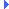FD.io VPP  v17.07-30-g839fa73 Vector Packet Processing
svm_fifo_segment.c File ReferenceInclude dependency graph for svm_fifo_segment.c:

Go to the source code of this file.

## Functions

static void preallocate_fifo_pairs (svm_fifo_segment_header_t *fsh, svm_fifo_segment_create_args_t *a)

int svm_fifo_segment_create (svm_fifo_segment_create_args_t *a)
(master) create an svm fifo segment More...

int svm_fifo_segment_create_process_private (svm_fifo_segment_create_args_t *a)
Create an svm fifo segment in process-private memory. More...

int svm_fifo_segment_attach (svm_fifo_segment_create_args_t *a)
(slave) attach to an svm fifo segment More...

void svm_fifo_segment_delete (svm_fifo_segment_private_t *s)

svm_fifo_tsvm_fifo_segment_alloc_fifo (svm_fifo_segment_private_t *s, u32 data_size_in_bytes, svm_fifo_segment_freelist_t list_index)

void svm_fifo_segment_free_fifo (svm_fifo_segment_private_t *s, svm_fifo_t *f, svm_fifo_segment_freelist_t list_index)

void svm_fifo_segment_init (u64 baseva, u32 timeout_in_seconds)

u32 svm_fifo_segment_index (svm_fifo_segment_private_t *s)

## Variables

svm_fifo_segment_main_t svm_fifo_segment_main

## Function Documentation

 static void preallocate_fifo_pairs ( svm_fifo_segment_header_t * fsh, svm_fifo_segment_create_args_t * a )
static

Definition at line 21 of file svm_fifo_segment.c.Here is the call graph for this function:Here is the caller graph for this function:

 svm_fifo_t* svm_fifo_segment_alloc_fifo ( svm_fifo_segment_private_t * s, u32 data_size_in_bytes, svm_fifo_segment_freelist_t list_index )

Definition at line 221 of file svm_fifo_segment.c.Here is the call graph for this function:Here is the caller graph for this function:

 int svm_fifo_segment_attach ( svm_fifo_segment_create_args_t * a )

(slave) attach to an svm fifo segment

Definition at line 178 of file svm_fifo_segment.c.Here is the call graph for this function:

 int svm_fifo_segment_create ( svm_fifo_segment_create_args_t * a )

(master) create an svm fifo segment

Definition at line 87 of file svm_fifo_segment.c.Here is the call graph for this function:Here is the caller graph for this function:

 int svm_fifo_segment_create_process_private ( svm_fifo_segment_create_args_t * a )

Create an svm fifo segment in process-private memory.

Definition at line 138 of file svm_fifo_segment.c.Here is the call graph for this function:Here is the caller graph for this function:

 void svm_fifo_segment_delete ( svm_fifo_segment_private_t * s )

Definition at line 213 of file svm_fifo_segment.c.Here is the call graph for this function:Here is the caller graph for this function:

 void svm_fifo_segment_free_fifo ( svm_fifo_segment_private_t * s, svm_fifo_t * f, svm_fifo_segment_freelist_t list_index )

Definition at line 289 of file svm_fifo_segment.c.Here is the call graph for this function:Here is the caller graph for this function:

 u32 svm_fifo_segment_index ( svm_fifo_segment_private_t * s )

Definition at line 341 of file svm_fifo_segment.c.

 void svm_fifo_segment_init ( u64 baseva, u32 timeout_in_seconds )

Definition at line 332 of file svm_fifo_segment.c.Here is the caller graph for this function:

## Variable Documentation

 svm_fifo_segment_main_t svm_fifo_segment_main

Definition at line 18 of file svm_fifo_segment.c.